index: click on a letter A B C D E F G H I J K L M N O P Q R S T U V W X Y Z A to Z index index: subject areas numbers & symbols sets, logic, proofs geometry algebra trigonometry advanced algebra & pre-calculus calculus advanced topics probability & statistics real world applications multimedia entrieswww.mathwords.com about mathwords website feedback

Integral Table

For the following, the letters a, b, n, and C represent constants.

Note: Most of the following integral entries are written for indefinite integrals, but they also apply to definite integrals.

 Basic Forms 1., where n ≠ –1 2.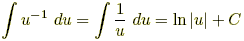3.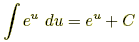4., where a > 0 and a ≠ 1 5.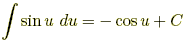6.7.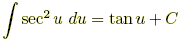8.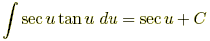9.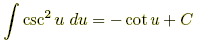10.11., where F(u) is an antiderivative of f(u) Other Basic Trig and Inverse Trig Forms 12.13.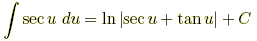14.15.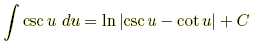16.17.for a > 0 18.19.for a > 0 20.for u > 1 21.for u > a > 0

 Basic Rational Forms 22.for a > 0 23.for a > 0 Trig Forms 24.25.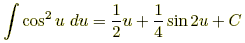26.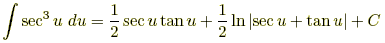Inverse Trig Forms 27.28.29.30.31.for u > 1 32.for u > 1 Forms Involving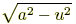33.for a > 0 Forms Involving34.Forms Involving35.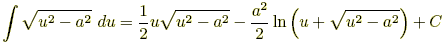for u > a > 0 Forms Involving  au + b 36.37.Exponential Forms 38.39.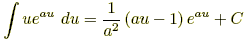40.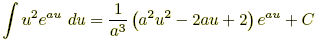41.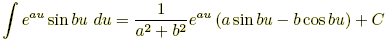42.Logarithmic Forms 43.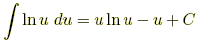Definite Integrals 44.